﻿
Reach Us+44-1904-929220
Longitudinal Imperfections on Thin Walled Cylindrical Shells

Journal of Civil & Environmental EngineeringOpen Access

All submissions of the EM system will be redirected to Online Manuscript Submission System. Authors are requested to submit articles directly to Online Manuscript Submission System of respective journal.

Longitudinal Imperfections on Thin Walled Cylindrical Shells

Mahyar Maali1, Abdulkadir Cuneyt Aydın1*, Hossein Showkati2, Seied Mahdi Fatemi3 and Merve Sagıroglu4
1Department of Civil Engineering, Faculty of Engineering, Ataturk University, Erzurum, Turkey
2Department of Civil Engineering, Urmia University, Urmia, Iran
3Department of Civil Engineering, Islamic Azad University, Maragheh Branch, Iran
4Department of Civil Engineering, Faculty of Engineering, Erzurum Technical University, Erzurum, Turkey
*Corresponding Author: Abdulkadir Cuneyt Aydın, Department of Civil Engineering, Faculty of Engineering, Ataturk University, Erzurum-25240, Turkey, Tel: +90 442 231 47 81, Email: [email protected]

Received Date: Jan 06, 2018 / Accepted Date: Apr 20, 2018 / Published Date: Apr 26, 2018

Abstract

Buckling and post-buckling are among the most important failure factors in thin walled structures. The loadcarrying behavior of cylindrical thin-walled shell structures under external pressure load is strongly dependent upon the nature and magnitude of the initial imperfections. These imperfections are invariably caused by an assortment of manufacturing processes like installing or welding. One of the most important imperfections caused by welding that has been reported to have an essential detrimental effect on the buckling resistance of these shells under external pressure load is longitudinal imperfections. Buckling and post buckling capacity of the shells depend on the H/R and t/ R ratios (H the height, R the radius and t the thickness of a cylindrical shell). The present work discusses the finiteelement models labeled as SS (Shallow Slim), DS (Deep Slim), ST (Shallow Thick) and DT (Deep Thick). The samples of first group are modiﬁed to include a line longitudinal imperfection, amplitudes of 0.5t, 1t, 2t, 3t, 4t and 8t in depth (t is the thickness of cylindrical shell). The results presented are in agreement with international codes and theories concerning buckling.

Keywords: Buckling; Cylindrical shells; Longitudinal imperfection; Non-linear analysis; Perfect model

Notations

E: Young`s modulus; R: Radius of cylinder; L: Height of cylinder; t: Thickness of cylinder; n: Number of approximate waves

Introduction

Buckling and collapse are two structures of thin-walled cylindrical shells. Buckling of a cylindrical shell depends on the scores of variables, for example, the geometric properties, the material properties and the type of the applied load. Thin-walled circular cylindrical shells are very common in civil engineering applications, such as tanks, silos, offshore and marine structures, ship industrial chemical plants [1-4]. Rolling and construction have much effect on buckling and postbuckling capacity of the cylindrical shells. The buckling capacity of the cylindrical shell depends greatly on the following two geometric rations: H/R (height to the radius of shell), and the slenderness t/R (thickness to the radius of shell) . There is a lot of literature devoted to the analysis of geometrically imperfect cylindrical shells. In 1995, Showkati and Ansourian  investigated the influence of primary boundary conditions on the buckling of shallow cylindrical shells under uniform external pressure . Donnell calculated the buckling load for a cylindrical shell and obtaining a theoretical load on the cylindrical shell under hydrostatic pressure . In 2001, Pircher et al.  studied the shape of circumferential weld-induced imperfections in thin–walled steel silos and tanks, and introduced several shapes of circumferential imperfections, which occurs in real conditions. Many researchers studied the buckling resistance of cylindrical shells through nonlinear finite-element methods. Hornug and Saal  searched on real-size tanks to examine the effects of imperfections on the buckling load of cylindrical shells. Schneider and Brede  studied the effects of geometric imperfections on the buckling resistance of cylindrical shells. Maali et al.  studied the buckling behavior of conical shells and showed the stiffening effect of weld-induced imperfections on the buckling strength. In 2013, Fatemi et al.  conducted experiments on imperfect cylindrical shells under uniform external pressure and showed the detrimental effects on the buckling of weld-induced geometric imperfections. Niloufari et al.  conducted experiments on imperfect steel tanks under hydrostatic pressure and showed the detrimental effects on buckling and post buckling of weld-induced geometric imperfections. Additionally, Eurocode 3, ECCS and DINI18800 [12-18] have all set limitations for rolling- and welding-induced imperfections.

In this study, not only presents the longitudinal overall imperfection, which is same circumferential imperfection in Picher’s paper , but also presents the effects of imperfection on the buckling of circular cylindrical shells under external uniform pressure with different H/R and R/t ratios.

Materials and Methods

The present study considers 28 cylindrical shells in four groups with different H/R and t/R ratios. All models contained one perfect model with the remaining models having imperfections with amplitudes of t (t the thickness of cylindrical shell). Average yield and failure stresses were obtained 194.2MPa and 325.5MPa, respectively. Young’s modulus calculated as 200GPa and Poisson’s ratio was obtained as 0.28 . All models were simply supported and analyzed by ABAQUS software. The results of the buckling were not only compared to the results reported in previous and international codes, but also compared with the perfect model.

Size and imperfect shape

According to previous research on thin–walled cylindrical shells, and also international codes have all set limitation for rolling and welding induced imperfection [12-18]. We decided to choose the different thickness-to-radius ratio (t/R) within the range of 0.001- 0.0033 .

Four groups of models were tested for this study. The first group is SS (Shallow Slim labeled specimens labeled as SSP, SS0.5, SS1, SS2, SS3, SS4, and SS8. The second group of specimens is DS (Deep Slim) ones labeled as DSP, DS0.5, DS1, DS2, DS3, DS4, and DS8. The third group labeled ST (Shallow Thick) labeled as STP, ST0.5, ST1, ST2, ST3, ST4, and ST8. The fourth group is named as DT (Deep Thick) with the specimens labeled as DTP, DT0.5, DT1, DT2, DT3, DCT4 and DT8. Table 1 provides the detailed geometry and slenderness ratios of the specimens. All models were considered as simply supported and analyzed by ABAQUS software in accordance with the past research [2-7]. Therefore, in this study, we selected good geometric imperfection, which occurs in real conditions. The imperfection type is as shown in Figure 1.

Model R (mm) t (mm) H/R t/R Mode Model R (mm) t (mm) H/R t/R Mode
SSP 300 0.5 1 0.002 Perfect STP 300 0.6 1 0 Perfect
SS0.5 300 0.5 1 0.002 0.5t* ST0.5 300 0.6 1 0 0.5t
SS1 300 0.5 1 0.002 1t ST1 300 0.6 1 0 1t
SS2 300 0.5 1 0.002 2t ST2 300 0.6 1 0 2t
SS3 300 0.5 1 0.002 3t ST3 300 0.6 1 0 3t
SS4 300 0.5 1 0.002 4t ST4 300 0.6 1 0 4t
SS8 300 0.5 1 0.002 8t ST8 300 0.6 1 0 8t
DSP 300 0.5 1.5 0.002 Perfect DTP 300 0.6 1.5 0 Perfect
DS0.5 300 0.5 1.5 0.002 0.5t DT0.5 300 0.6 1.5 0 0.5t
DS1 300 0.5 1.5 0.002 1t DT1 300 0.6 1.5 0 1t
DS2 300 0.5 1.5 0.002 2t DT2 300 0.6 1.5 0 2t
DS3 300 0.5 1.5 0.002 3t DT3 300 0.6 1.5 0 3t
DS4 300 0.5 1.5 0.002 4t DT4 300 0.6 1.5 0 4t
DS8 300 0.5 1.5 0.002 8t DT8 300 0.6 1.5 0 8t

*Imperfection depth

Table 1: Dimensions and geometric ratios of the specimens.

The imperfection is composed of a four quarter–circle compound with a radius of Bt (Bt is the depth of the imperfection).

The Buckle and Riks analyses

The buckling load can be obtained by the liner (Eigen value) and nonlinear analyses in the Abaqus software. As a result of static linear analysis and internal forces, the stiffness matrix of the structure and the Eigen value mode can be obtained from the equilibrium equations. The linear method simply calculates the overall distribution of stress and the relative displacement. Furthermore, the buckling shape mode and factor load offered by the linear method can be obtained . The nonlinear methods calculate the real displacement and stress. Therefore, the Arc length (Riks) method is used, as a proper method for solving the nonlinear buckling problem and to determine the load-displacement path within a more realistic way. By this way, the main characteristics are considered as nonlinear, unlike the Eigen value method. The most important point in this method is to shorten the maximum arc length, when the load amounts become negative and the analyses become unstable after arriving to bifurcation point.

Theoretical analyses

The analytical solutions given here ignore the effect of pre-buckling rotations,and assume the cylinder as free to stretch longitudinally. The simplified version of the Donnell Stability equations (1) in coupled form and for uniform pressure q is :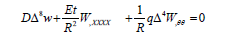……....(1)

Where D = Et3/12 (1-υ2), w=radial deflection, x= meridional distance and θ= circumferential angle. Symmetry considerations imply periodicity and the general solution in terms of the four constants of integration is given by: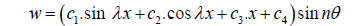…… (2)

Where λ = mπ/Land m, n=1, 2, 3, … . The boundary conditions are used to solve the four constants. The case that is amenable to analytical solution is S-S, which corresponds to radial restraint at the two ends. Substitution of the conditions of zero radial deflection and moment at the ends and minimization of the buckling solution with respect to the wave numbers m and n leads to the solution m=1 and: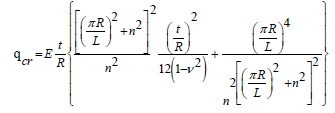…… (3)

This critical pressure is a function of n4 obtained following minimization of qcr as: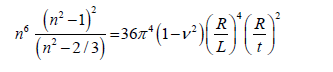…… (4)

Some simplification is possible when n2 is much greater than 1, when n becomes: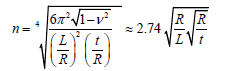…… (5)

Except in the case of classical simple supports and a limited geometry, it is not generally possible to find analytical solutions for n. In addition, a full numerical investigation of n under general boundary conditions has not been noted throughout the literature except possibly for the case of clamped supports at both ends. The case of elastic end restraint is not considered throughout this paper, although this will be the subject of a subsequent paper .

The follower load effects have not been taken into account in this investigation. It has been reported that, in long and thick cylinders, the effect is to reduce the critical pressure by a few percent when compared with solutions that consider a constant load direction. Long and thick cylinders are also less imperfection sensitive. The range of parameters is considered in this paper, such that the neglect of the follower load effect leads only to very small errors except for the case where n=2. The cases n=0 and n=1 are special cases of axisymmetric, beam-like or torsional buckling. In the case of external pressure loading, n has a minimum value of 2, which is the ovalization mode in very long, or long and thick cylinders, or short cylinders with special boundary conditions. In a mathematical treatment of buckling, when the pressure is minimized with respect to n, n is assumed to be a continuous variable, although is in fact an integer value. In this case, the waves are of the same length, while, under non-uniform loading, the modal pattern is not regular for wind pressure loading .

For other boundary conditions, numerical methods are generally required. When the geometric parameter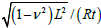obtained as one (very short cylinders), the behavior is like a long, flat, rectangular plate in uniaxial compression for most boundary conditions. When the pressure is ‘hydrostatic’, the equivalent plate is bi-axially compressed. The geometric analogy is that the plate thickness is also t, the length a; corresponds to 2πR, and the width b to L. The results for these very short cylinders are not reproduced here, but elsewhere .

The critical buckling load of thin-walled cylindrical shells is estimated by equation (6), which was obtained from Donnell's exact solution with some simplifications. This is offered for an ideal geometric form .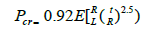……(6)

Where E is the Young’s modulus, t is the thickness, R is the radius and L is the height. Table 2 presents the results from the Donnell equation and the number of circumferential buckling waves, calculated through approximate equation (5) for the perfect models.

Model SSP DSP STP DTP
Pcr - Eq. 6 (kPa) 20.9 13.9 32.9 21.9
Eq.5 Approx. number of waves 14 11 13 10

Table 2: Results of the critical buckling load and wave equation.

Finite-element mesh

We select S4R element and 6 degrees of freedom for each point, which is especially shell by past research and Abaqus software laboratory. It's designed for linear and nonlinear analyses and it is a useful tool for large deformities [20,21]. In this study, the SSP and DTP Models are selected, and the ideal mesh is obtained through linear buckling load analyses when an ideal mesh is obtained for SSP and DTP models, that mesh can be better for all models. According to analysis of buckling load, a proper mesh is obtained with an approximate global size as 0.005. Furthermore, we selected one point in each 5mm on cylindrical shell for mesh section. Figures 2 and 3 and Tables 3 and 4 present the selection of the mesh for SSP and DTP models. In addition, we shortened distance meshes to half of another point distance in imperfection location. Thus, the accuracy level of the analysis is increased. We also used sweep technical mesh with quad-dominated shape in this study. This technique causes coordinating and adapting of the structure to meshing formed.

Approximate global size Eq. 6 Linear buckling load obtained by Abaqus (KPa) (PFEA)  (PFEA)/ (Pcr)
(kPa)Pcr
0.03 20.86 40.872 1.96
0.02 20.86 34.207 1.64
0.01 20.86 30.755 1.47
0.009 20.86 30.554 1.46
0.007 20.86 30.194 1.45
0.005 20.86 29.905 1.43
0.003 20.86 29.716 1.43

Table 3: Results of the buckling load for SSP model.

Approximate global size Eq. 6 (kPa)Pcr Linear buckling load obtained by Abaqus (KPa) (PFEA)  (PFEA)/ (Pcr)
0.03 21.94 38.491 1.75
0.02 21.94 34.371 1.57
0.01 21.94 32.214 1.47
0.009 21.94 32.084 1.46
0.007 21.94 31.867 1.45
0.005 21.94 31.707 1.44
0.003 21.94 31.602 1.44

Table 4: Results of the buckling load for DTP model.

All models analyzed under uniform external pressure load and analyzed by ABAQUS software. All models were considered simply supported while they assumed the third displacement components are closed and the third rotation components are opened.

Results of Buckle Analysis (Linear Analysis)

As mentioned in most articles where cylindrical shells under pressure load have been investigated, we used in equation 6 for normalizing the result of a perfect model. Table 2 presents the results of the Donnell equation for the Abaqus models. There are no equations for imperfection models. Furthermore, we have done linear analysis to find wave numbers for each group. Tables 5-8 present the linear analysis for perfect and imperfect models and wave number for each model. Figures 4-7 present the result of linear analysis and wave buckling for each group.

Model SSP SS0.5 SS1 SS2 SS3 SS4 SS8
Linear (buckle) (KPa) 29.91 29.887 29.82 29.43 28.92 29.43 29.93
Percent to perfect (%) _ -0.06 -0.295 -1.631 -3.42 -1.63 0.094
Percent to Eq. 6 (%) 30.25 30.22 30.04 29.11 27.86 29.11 30.31
Axial wave 1 1 1 1 1 1 1
Circumferential wave 16 16 16 16 16 16 16

Table 5: Results of the linear analysis of SS models.

Model DSP DS0.5 DS1 DS2 DS3 DS4 DS8
Linear (buckle) (KPa) 20.09 20.076 20.05 19.95 19.7 19.39 29.93
Percent to perfect (%) _ -0.045 -0.154 -0.671 -1.98 -3.595 32.9
Percent to Eq. 6 (%) 30.74 30.71 30.64 30.28 29.37 28.25 53.53
Axial wave 1 1 1 1 1 1 1
Circumferential wave 13 13 13 13 13 13 13

Table 6: Results of the linear analysis of DS models.

Model STP ST0.5 ST1 ST2 ST3 ST4 ST8
Linear (buckle) (KPa) 46.8 46.76 46.66 45.95 45.41 46.78 47
Percent to perfect (%) _ -0.068 -0.302 -1.857 -3.06 -0.045 0.41
Percent to Eq. 6 (%) 29.67 29.63 29.46 28.37 27.52 29.64 30
Axial wave 1 1 1 1 1 1 1
Circumferential wave 15 15 15 15 15 15 15

Table 7: Results of the linear analysis of ST models.

Model DTP DT0.5 DT1 DT2 DT3 DT4 DT8
Linear (buckle) (KPa) 31.71 31.69 31.63 31.35 30.77 30.38 31.8
Percent to perfect (%) - -0.054 -0.237 -1.145 -3.062 -4.36 0.32
Percent to Eq. 6 (%) 30.8 30.76 30.64 30.01 28.68 27.78 31
Axial wave 1 1 1 1 1 1 1
Circumferential wave 12 12 12 12 12 12 12

Table 8: Results of the linear analysis of DT models.

Comparison between the results of the linear Analysis of the SS and DS groups of models with the ratio of t/R equal to 0.0016

The results of buckling analysis were 1.54 times larger than the result of the Donnell theory in the perfect models (without imperfection) which the result of linear analysis is inconsistent with the result of the Donnell theory and the result of linear analysis are conservative. Therefore, it is fitting that we use of the nonlinear analysis method (Riks method) for investigation buckling loads of cylindrical shells.

The theoretical buckling loads obtained from the Donnell theory from the SS and DS group models are in the range between 27-54 percent less than the results of linear analysis. Therefore, if we used linear analysis for investigation buckling load of perfect and imperfect cylindrical shell. The result of linear analysis is greater than 1.54 times the results from the Donnell theory.

According to Tables 5 and 6, the imperfection depth is greater. The imperfect models ration to perfect models is the same as a stiffener. For example, the result of the Buckle analysis for SSP, SS2 and SS8 models is 29.905 KPa, 29.425 KPa and 29.933 KPa, respectively. These show that the buckling capacity is increased, and 8t imperfection can be used as a stiffener for cylindrical shells. As shown in Figures 4 and 5, axial number waves are the same as each other. The number of the wave is 1 and circumferential wave numbers are 16 and 13 for the SS and DS models, respectively. Figures 4 and 5 show the circumferential waves are disappeared between ranges of 2t- 4t imperfections. Then, those are appearing in 8t imperfection, because of the 8t imperfection acts as a stiffener. There is a wave on an imperfection section in all models without SS8 and DS8 models are the same as a stiffener. We obtained the number of circumferential waves by 5 equations, which are shown in Table 2. The exact number of waves can be obtained by addition of 5th and 2nd equations.

Comparison between the results of the linear Analysis of the ST and DT groups of models with the ratio of t/R equal to 0.002

The results of buckling analysis showed 1.32 times larger than the result from the Donnell theory in the perfect models (without imperfection), where the result of linear analysis is inconsistent with the result from the Donnell theory. The results of linear analysis are conservative. Therefore, the nonlinear analysis method (Riks method) is a proper way for investigating buckling loads of cylindrical shells.

The theoretical buckling loads, obtained with the Donnell theory from ST and DT group models, are in the range between 27-32 percent less than the linear analysis. Therefore, the linear results are 1.32 times greater than the Donnell theory, for the investigation of perfect and imperfect cylindrical shells buckling load.

According to Tables 7 and 8, imperfection models are acted as stiffened ones. For example, the result of the Buckle analysis for DTP, DT2 and DT8 models is 31.707 KPa, 31.348 KPa and 31.810 KPa, respectively. These show that the buckling capacity is increased. Thus, 8t imperfection can be used as a stiffener, and it is useful for cylindrical shells. As seen in Figures 6 and 7, axial number waves are the same as each other, and the wave number is 1 and circumferential wave numbers are 15 and 12 for the SS and DS models, respectively. Figures 4 and 5 show the circumferential waves, which are disappeared between ranges of 2t-4t imperfections. Then, those are appearing in 8t imperfection. Because of the 8t imperfection, it acts as a stiffener. There is a wave on an imperfection section in all models except ST8 and DT8 models, which are the same as a stiffener. We obtained the number of circumferential waves by the 5th equations which are shown in Table 2. If we compare the results of equation 5 with imperfection models, we should add equation 5 to 2 to obtain correct number waves.

Comparison between the result of the linear analysis of all groups of models with the ratio of t/R equal to 0.0016 and 0.002

While the values of t/R between 0.0016 and 0.002, the buckling loads obtained from the linear theory is 1.54 times greater than the Dunnel theory. The number of circumferential waves can be obtained by adding 5th and 2nd equations.

However, the imperfection depth is greater. The imperfections act as a stiffener in all groups. For example, 8t imperfection is as a stiffener, because the buckling load capacities in 8t imperfection models are greater than the other models in all groups. Furthermore, the height of the cylindrical (H) is higher. So, the buckling load capacity is low. The 0.5t and 1t type imperfections are weaker and their effects low. Thus, 0.5t and 1t type imperfections are neutral, and those are the same as the perfect models. The critical buckling load capacity occurs between 2t-3t type imperfections, which disappear in the range of all groups.

Results of Riks Analysis (Non-linear)

Non-linear analysis of the SS and DS groups of models with the ratio of t/R equal to 0.0016

Table 9 presents the results of initial and collapse buckling to the Donnell theory for the perfect models. The comparison between the results of Eq. (6) and the results of the t/R=0.0016 models reveal that the obtained initial buckling load of the Riks analysis is 26%-36% greater than the Donnell theory. The buckling load for the collapse is 30%-36% greater than the Donnell theory. Therefore, if we want to obtain the non-linear buckling load from the Donnell theory for t/R=0.0016, we should multiply the Donnell theory with 1.36.

Model Initial Buckling (kPa) (FEA) Collapse (kPa) (FEA) Percent initial buckling to Perfect (%) Percent collapse to Perfect (%) Percent initial buckling to Eq. 6 (%) Percent collapse to Eq. 6 (%) Post-Buckling Percent collapse to Initial (%)
SSP 32.38 32.6 --- --- 35.58 36.01 0.67
SS0.5 27.95 29.7 -15.85 -9.76 -- -- 5.89
SS1 24.5 28.65 -32.16 -13.78 -- -- 14.48

Table 9: Results of the non-linear analysis for t/R equal to 0.0016.

Table 9, Figures 8 and 9 present the results of the initial buckling by Riks analysis for SS and DS models. Although the imperfection depth is greater, the imperfections act weaker, where a negative effect is occurred in the SS4 and DS4 which present initial buckling to the perfect model are -196.52% and -159.89% for SS4 and DS4, respectively. Table 9 shows the 8t type imperfection. It acts as a stiffener than to the other imperfections. However, the imperfection depth is greater; the collapse load is decreasing until 4t type imperfection. After that, the collapse load is suddenly increased. Thus, the models have a big imperfection effect such as a stiffener. Conversely, all models showed the post-buckling phenomenon, while after initial buckling a length path obtained, until the collapse occurred by buckling. For example, in the SS8 model, the initial buckling load is 15.10 KPa, and the collapse load is 83.70 KPa. The collapse load is 4.7 times greater than the initial buckling load. Table 9 shows the 8t type imperfection acts as a stiffener more than to the other ones.

Figures 10 and 11 show the buckling effect started from the imperfection located and continued by the cylindrical shell. Figures show, which ˅ and ˄ shapes causes lift the edge, and end of analysis. The 8t type imperfection models are resisted against buckling as seen in Figures 10 and 11.

Non-linear analysis of the ST and DT groups of models with the ratio of t/R equal to 0.002

Table 10 presents the initial buckling and collapse loads’ comparison according to the Donnell theory. The obtained initial buckling and the collapse load of the Riks analysis is 25% to 35%, and 30% to 36% greater than the Donnell theory, respectively. Table 10, Figures 12 and 13 present the results of the initial buckling by Riks analysis for ST and DT models. When the imperfection depth increases in the SS4 and DS4 models, negative effects occurred and the imperfection acts as weakener. The initial buckling loads are -249.09% and -175.12% for the ST4 and DT4 models, respectively. Table 10 shows the 8t imperfection model, which acts as a stiffener than to other imperfections. However, when the imperfection depth increases, the collapse load decreases until 4t imperfection model. Then, the models have greater imperfection resulted as a stiffener. The post-buckling phenomenon obtained in all models. After the initial buckling load, a length path occurred until the collapse for all models. For example, in the DT8 model, the initial buckling load and the collapse load is 13.92 KPa, and 50.42 KPa, respectively. The collapse load is 3.6 times greater than the initial buckling load. Table 10 shows the 8t imperfection model, and it acts as a stiffener than to other imperfections. Figures 14 and 15 show the buckling effect, which ˅ and ˄ shapes cause lift the edge and end of analysis. The 8t imperfection models are stabil and resistant against buckling, as seen in Figures 14 and 15.

Model Initial Buckling (kPa) (FEA) Collapse (kPa) (FEA) Percent initial buckling to Perfect (%) Percent collapse to Perfect (%) Percent initial buckling to Eq. 6 (%) Percent collapse to Eq. 6 (%) Post-Buckling Percent collapse to Initial (%)
STP 50.05 51.78 --- --- 34.46 36.44 3.34
ST0.5 41 46.5 -22.07 -11.35 --- --- 11.83
ST1 34.3 42.985 -45.92 -20.46 --- --- 25.32

Table 10: Results of the non-linear analysis for t/R equal to 0.002.

Non-linear analysis of all groups with t/R=0.0016 and t/ R=0.002

Whatever the imperfection depth is greater, imperfections act as weakeners until the 4t imperfection. It afterwards acts as a stiffener in the other three groups. The weakest effect occurred in the 4t imperfection model from all groups. When the height of the cylinder increased, the buckling load capacity is decreased in each group. For example, the initial buckling load of the STP model is equal to 50.4 KPa. The initial buckling load of DTP model is equal to 29.30 KPa for variable heights. When the thickness of cylinder increased, the buckling load capacity is increased in each group. For example, the initial buckling of the STP model is equal to 19.05 KPa, while the initial buckling load of DTP is equal to 29.30 KPa with same thickness. The nonlinear buckling load can be obtained by multiplying with 1.36 the results from the Donnell theory for t/R=0.002 and t/R=0.0016 models.

There is post-buckling phenomenon and a length path after initial buckling load until the collapse in all models. The collapse load is 3.6 times greater than the initial buckling for the t/R=0.002 models and 4.7 times for t/R=0.0016 models.

The buckling effects started with imperfection locate and continue to the cylindrical shell. Figs showed which ˅ and ˄ shapes causes lift the edge and end of analysis also 8t imperfection models are stability and resistant to buckling.

Discussion

The ABAQUS analyses and experimental results of Fatemi et al.’s work

In 2013, the authors have conducted tests on SS, DS, ST and DT models with perfect, the 4t and 8t imperfection models  and compared the results with ABAQUS models (Table 11). It should be noted that all models were supported in ABAQUS models, while all models were supported in which a radial constraint at the edges was the only boundary condition in experimental models.

Model Experimental Collapse load  (kPa) ABAQUS collapse load (kPa) Experimental waves ABAQUS waves ( linear waves) Percent ABAQUS to Experimental (%)
SCSP 28.57 32.6 10 16 12.28
SCS4 27.95 27.8 10 16 -0.53
SCS8 33.975 83.7 10 16 59.41
DCSP 14.125 19.95 8 13 29.2
DCS4 17.575 18.75 7 13 6.3
DCS8 17.825 42.2 9 13 57.76
SCTP 38.7 51.7 10 15 25.14
SCT4 29.3 44.47 9 15 34.11
SCT8 30.6 46 10 15 33.48
DCTP 18.375 31.4 8 12 41.48
DCT4 19.7 34 8 12 42.06
DCT8 20.425 50.42 9 12 59.49

Table 11: Comparison between ABAQUS analyses and experimental results of Fatemi’s works.

Table 11 presents the results of the buckling loads from Riks analysis and experimental tests  of Fatemi et al.’s work. The boundary condition has many effects in buckling loads and number of circumferential waves. Table 11 shows the models with simple support, which have been greater buckling loads than the models considered as simply supported with a radial constraint at the edges. Within the ABAQUS analyses, the simple support with a radial constraint at the edges in experimental were -1% to 60%, and the models with simple support have a greater number of circumferential waves than models considered with a radial constraint at the edges. The number of circumferential waves obtained from ABAQUS analyses and experiments, the number of waves were between 33% and 67%.

Figure 16 of the experimental model is the same as (Figures 4-7, 10, 11, 14, 15). As seen, the buckling effect is started with the imperfection located and continued to the cylindrical shell. The failure shapes of cylindrical waves are the same as ˅ and ˄ which ˅ and ˄ failure shapes cause lift of the edge and end of analysis or tests .

Figure 16: Post-buckling behavior torsion ˅ and ˄ shaped failure mode for experimental models.

Geometrical imperfections tolerated by the standards

International codes (such as Eurocode 3, ECCS and DIN 18800- 4) for designing thin-walled shells simply limit the permitted deformations and disturbances during the welding and construction of conical shells (Table 4). Various methods proposed for measuring the magnitude of the initial imperfection depth on the shell’s skin in the longitudinal direction include the following:

lg= 4(r/t)0.5

lg=25t ≤ 500 mm at welds for prENV 1993-1-6 (2007): Eurocode 3 

lr=4(r/t)0.5 ≤ 0.95 times the distance of the welds

lr=25t at welds for ECCS (2008) 

lmx=4(r/t)0.5 ≤ 2000 mm

lmx ≤ 500 mm at welds for DIN 18800 

In these equations, r is the middle surface of the conical shell, t is the thickness of the cone and lmx, lr and lg are all the longitudinal imperfections created circumferentially. The mx, r and g indexes in different codes represent the same length in various forms. Table 12 demonstrates the exact location of this dimension. In the present study, the size of the dent tv and the length of the curve lmQ were designed and created with precise tools, so there is no need to compute the limitations of the above-mentioned formulas.

trtrtr
 Definition of the depth of initial dimples Tolerances according to different standards Depth of initial dimples in plates measured vertically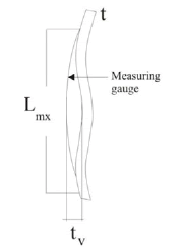DIN 18800-4 tr ˂1% of measuring length Lmx ESSC Rec {2008} L= Ir:tr=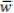tr ˂1% of measuring length Lmx pr ENV 1993-1-6 {2007} Lmx=Lg:tr= Δw0 class A:tr ≤0.006 Lmx class B:tr ≤0.010 Lmx class C:tr ≤0.016 Lmx Initial dimple of circumferential weld seam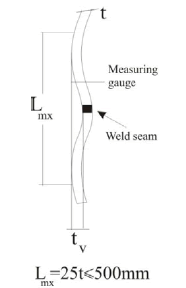DIN 18800-4 tr ˂1% of measuring length Lmx ESSCRec {2008} Lmx= Ir:tr=tr ˂1% of measuring length Lmx pr ENV 1993-1-6 {2007} Lmx=L:tr= Δw class A:tr ≤0.006 Lmx class B:tr ≤0.010 Lmx class C:tr ≤0.016 Lmx

Table 12: Geometric tolerances according to different standards.

The values of tv for the 0.5t, 1t, 2t, 3t, 4t and 8t models, where t is the thickness of the shell, were 0.25mm, 0.5mm, 1mm, 1.5mm, 2 mm and 4 mm for t=0.5 and were 0.3mm, 0.6mm, 1.2mm, 1.8mm, 2.4 mm and 4.8 mm for t=0.6, respectively. The values of lmQ related to 2t, 4t, 8t,12t, 16t and 32t were 1 mm, 2 mm, 4 mm, 6 mm, 8 mm and 16 mm for t=0.5 and were 1.2 mm, 2.4 mm, 4.8mm, 7.2 mm, 9.6 mm and 19.2 mm for t=0.6, respectively. The computation of tv and lmQ reveals that the specimen values far exceeded the amounts allowed by codes; in such cases, the structure cannot be used.

Calculation of the tv and lmQ reveals that the imposed tv exceeds the value allowed by the code and the structure should stop functioning. The codes do not refer to destruction of these structures. The Abaqus analyses results show that in some structures, imperfections strengthen the shell and, in some cases, increase the resistance as much as three times. In these cases, there is no need to stop using these structures and their function should continue with special care. The codes related to thin-walled shells have no references to studies referring to the postbuckling capacities of shells. The codes simply suggest not using such structures. This conclusion requires a great deal of investigation and thought .

Conclusion

This study discusses the buckling behavior of thin-walled conical shells. The 28 models with various imperfections with various amplitudes were loaded under external pressure. All models were analyzed by Abaqus software. The following results were concluded:

a) The number of circumferential waves in buckling analyses can be obtained by adding 5th and 2nd equations.

b) Axial waves are equal to one in all groups. There is always an axial wave in cylindrical shells with longitudinal imperfection.

c) When the imperfection depth is greater, imperfections act as a stiffener in all groups in linear analyses.

d) The height of the cylinder (H) is higher; the buckling load capacity is low in linear analyses.

e) The critical buckling load capacity occurred between the 2t and 3t imperfections, which disappeared in the range of all groups in linear analyses.

f) Whenever the imperfection depth is greater, imperfections act as weakener until the 4t imperfection, and afterwards act as a stiffener in the three groups in the nonlinear analyses.

g) The weakest effect occurred in the 4t imperfection in all groups in the nonlinear analyses.

h) When the height of the cylinder increased, the capacity buckling load decreased in each group. When the thickness of the cylinder increased, the buckling load capacity increased in each group in nonlinear analyses.

i) The nonlinear buckling load can be obtained from the Donnell theory for t/R=0.002 and t/R=0.0016 models, by multiplying the Donnell theory with 1.36.

j) There is post-buckling phenomenon in all models. The collapse load is 3.6 times greater than the initial buckling load for the t/R=0.002 models and 4.7 times for t/R=0.0016 models.

k) The buckling effects started with imperfection located and continued to the cylindrical shell. Figures 10-16 showed which ˅ and ˄ failure shapes cause lifting of the edge and end of analysis.

l) The models with simple support have greater number of circumferential waves than models, which considered simply supported with a radial constraint at the edges. Furthermore, the number of circumferential waves from Abaqus analyses and from the experiments was between 33% and 67%.

m) The codes related to thin-walled shells have no references about post-buckling capacities of shells; the codes simply suggest that such structures should not be used. This conclusion requires a great deal of investigation and thought.

References

Citation: Maali M, Aydın AC, Showkati H, Fatemi SM, Sagıroglu M (2018) Longitudinal Imperfections on Thin Walled Cylindrical Shells. J Civil Environ Eng 8:308. DOI: 10.4172/2165-784X.1000309

Copyright: © 2018 Maali M, et al. This is an open-access article distributed under the terms of the Creative Commons Attribution License, which permits unrestricted use, distribution, and reproduction in any medium, provided the original author and source are credited.

Select your language of interest to view the total content in your interested language

Recommended Journals
Viewmore
Article Usage
• Total views: 1130
• [From(publication date): 0-2018 - Jun 25, 2019]
• Breakdown by view type
• HTML page views: 1065Can't read the image? click here to refresh